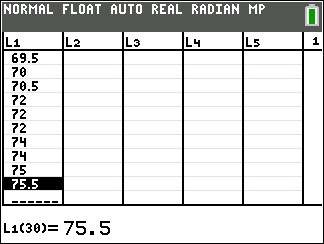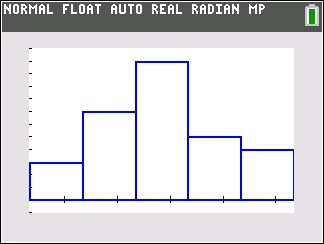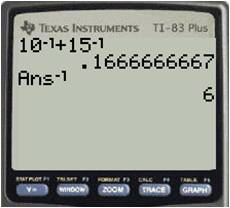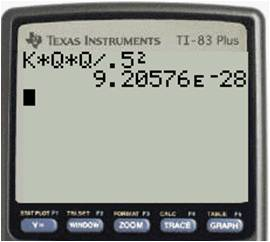## Making excellent histograms on your calculator

Your graphing calculator will make superb histograms from data you enter. If you have tried to do this, but your histograms don’t look the way you expect, follow this procedure. You have to tweak the x-min, x-max, and x-scale values to match your preferences. Let’s say that you have collected the following data (perhaps they are the heights of 30 people in your class):

61.0    61.5     62.0    65.0    65.5     65.5     66.0     66.0
66.5    66.5     67.0    67.0    67.5     68.0     68.5     68.5
69.0    69.0    69.0    69.5    69.5     70.0     70.5     72.0
72.0    72.0    74.0    74.0    75.0     75.5

 1. Determine the smallest class value and the class width. For this data set, I would display the data in five classes. We can set the smallest class value to 61, the largest class value to 76 and therefore the class width will be 3. 2. On your calculator, enter the data into a list. Here I’ve put it into L1.3. Go to STAT PLOT and turn one of the plots on. 4. Select the histogram type and if necessary specify the Xlist location.5. Press zoom and select 9 (ZoomStat). Your graph probably won’t look right, but we’ll fix that. 6. Press the WINDOW button and set Xmin= to the smallest class value, Xmax= to the largest class value, and Xscl= to the class width. You might also need to adjust the Ymin and Ymax values.7. Press GRAPH, and admire your excellent histogram.## Sum( and Seq( commands on your calculator

Can you find the sum of the following series?$\displaystyle \sum_{i=1}^{15}\dfrac{2n+1}{3n-2}$

This is neither an arithmetic nor a geometric series, so you don’t have a formula for it. This would be a tedious problem to do by hand. Fortunately, your graphing calculator can do these problems quickly and efficiently.

There are two functions you need to use on your calculator. The seq( command creates a sequence of terms based on a rule that you give. The sum( command adds together the terms in a sequence. Both functions are found on the LIST menu on your calculator. The seq( command is on the OPS submenu and the sum( command is on the MATH submenu.

To sum a series, you combine the two commands. If you have the new operating system on your calculator, it will prompt you for the entries when you select the seq( command. If you have the old operating system, you need to know the syntax for the command. The syntax for the series above is:

sum(seq((2x + 1)/(3x – 2),x,1,15))

Note that the seq( command has four parameters in the parentheses. From left to right, these are 1) the rule for the nth term of the sequence; 2) the variable name; 3) the first value of the variable; and 4) the final value of the variable. Now all you need to do is type this in to your calculator and let it do the crunching:

## Using the reciprocal key on your calculator

Your graphing calculator has a reciprocal key on it, designated as$x^{-1}$. It’s the easiest way to find the reciprocal of a number on your screen. Just press the key, and then Enter.

The reciprocal key is also a great way to do calculations of resistors in parallel or capacitors in series. For example, find the equivalent resistance of a circuit that has a 10 Ω and a 15 Ω resistor in parallel. Simply enter it this way into the calculator to find the answer is 6 Ω.## Storing constants on your graphing calculator

There are several fundamental constants in physics that you will use over and over. If you get tired of looking them up every time you need one for a calculation, consider storing them in your calculator. The graphing calculator makes it easy to store any value as one of the alphabet letters. It then remains in the calculator until you remove it.

To store a number, type it into the calculator, press the Store key (STO→), then select a letter with the ALPHA key. Then when you need the value, just type the letter on your calculator. Here are the constants I store in my calculator:

 Constant letter value Coulomb law constant k 8.99E9 Elementary charge q 1.60E-19 Planck’s constant h 6.63E-34 speed of light c 2.998E8 Universal gravitation constant G 6.67E-11

Other constants you might find useful to store are proton mass, electron mass, vacuum permittivity and permeability, and Boltzmann’s constant.

For example, to calculate the electrical repulsion force between two electrons that are fixed 0.5 m apart, use the equation$\displaystyle F = \frac{kq_{1}q_{2}}{r^2}.$

Because the values of$k$ and$q$ are already stored in my calculator, here’s the way this would appear when I crunch it: